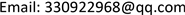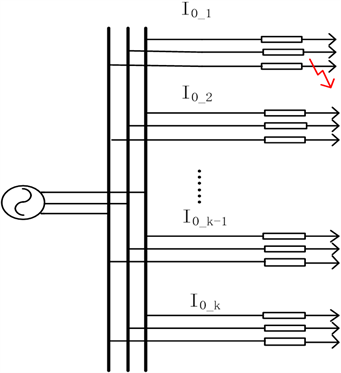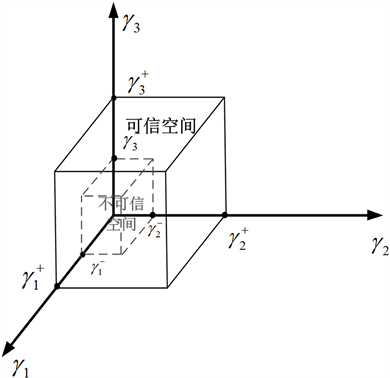﻿ 基于可信度评价指标的配电网选线方式综合决策 Comprehensive Decision of Distribution Network Line Selection Based on Credibility Evaluation Index

Journal of Electrical Engineering
Vol.05 No.04(2017), Article ID:23166,8 pages
10.12677/JEE.2017.54034

Comprehensive Decision of Distribution Network Line Selection Based on Credibility Evaluation Index

Mingbo Yang1, Getu Ma2, Sanjun Fan1, Yi Long2, Bo Wang1, Jinxin Ouyang2, Tiancai He2

1Guiyang Power Supply Bureau, Guizhou Power Grid Co, Guiyang Guizhou

2State Key Laboratory of Power Transmission Equipment & System Security and New Technology, School of Electrical Engineering, Chongqing University, ChongqingReceived: Dec. 6th, 2017; accepted: Dec. 19th, 2017; published: Dec. 26th, 2017ABSTRACT

The fault line selection technology of distribution system with small current grounding is the key to ensure the safe and reliable operation of distribution network. The research on the technology of line selection in distribution network mainly focuses on the principle of line selection. Due to the influence of the grid structure of distribution system, the way of neutral grounding, the outlet type, load and other factors, each method has its limitations. Currently, there is a lack of the study on comprehensive evaluation methods of line selection. In this paper, three different line selection methods, including zero-sequence current amplitude method, harmonic method and zero-sequence current active component method, are used to quantitatively analyze the influencing factors of each line selection method. The credibility evaluation index of different methods is defined and classified according to the credibility level. The comprehensive evaluation index is established through the construction of Euclidean distance quantitative index evaluation system, and the comprehensive selection of line selection method based on credibility evaluation is given. Finally, an example is given to verify the feasibility and practicability of this method.

Keywords:Distribution Network, Line Selection Technology, Comprehensive Decision, Credibility Evaluation Index

1贵州电网有限责任公司贵阳供电局，贵州 贵阳

2输配电装备及系统安全与新技术国家重点实验室(重庆大学电气工程学院)，重庆Copyright © 2017 by authors and Hans Publishers Inc.1. 引言

2. 选线可信度指标

2.1. 零序电流幅值比较法

${I}_{0_j}=\mathrm{max}\left({I}_{0_i},i=0~k\right)$ (1)

${\gamma }_{1}=\frac{{I}_{0_j}-{I}_{0_avg}}{{I}_{0_j}}×100%$ (2)

${I}_{0_avg}=\frac{\underset{i=1}{\overset{k}{\sum }}{I}_{0_i}}{k}$ (3)

2.2. 谐波法

${\gamma }_{2}=\frac{{{I}^{\prime }}_{0\left(5\right)}-{I}_{\left(5\right)}}{{{I}^{\prime }}_{0\left(5\right)}}×100%$ (4)Figure 1. Distribution system failure diagram

2.3. 零序电流有功分量方向法

${\gamma }_{3}=\mathrm{cos}\left(\angle {\theta }_{0}+\text{π}\right)$ (5)

3. 基于欧式距离综合评价模型

${U}_{\gamma }=\left({\gamma }_{1},{\gamma }_{2},{\gamma }_{3}\right)$ (6)

${\gamma }_{n}^{+},{\gamma }_{n}^{-}\left(n=1,2,3\right)$ 分别表示零序电流比幅法、谐波法、零序电流有功分量法对应指标理想值与可接受下限。当指标达到理想值时，表示该选线方式可满足可信度要求，能够正确选线，当指标跌落至可接受下限之下时，表示该选线方式无法满足可信度要求。根据大量仿真经验与实际工程数据，本文中 ${\gamma }_{n}^{+}$${\gamma }_{n}^{-}$ 取值如表1所示。Table 1. Route selection method credibility index acceptable lower limitFigure 2. Line selection confidence space

$CE{P}_{1}=\left({\gamma }_{1}^{+},{\gamma }_{2}^{-},{\gamma }_{3}^{-}\right)$ (7)

1) 针对指标LSCI_1，计算其与置信极值点欧式几何距离为：

${L}_{1}=\sqrt{{\left({\gamma }_{1}-{\gamma }_{\text{1}}^{\text{+}}\right)}^{2}+{\left({\gamma }_{2}-{\gamma }_{2}^{-}\right)}^{2}+{\left({\gamma }_{3}-{\gamma }_{3}^{-}\right)}^{2}}$ (8)

2) 针对指标LSCI_2，计算其与置信极值点的欧式几何距离为：

${L}_{2}=\sqrt{{\left({\gamma }_{1}-{\gamma }_{1}^{-}\right)}^{2}+{\left({\gamma }_{2}-{\gamma }_{\text{2}}^{\text{+}}\right)}^{2}+{\left({\gamma }_{3}-{\gamma }_{3}^{-}\right)}^{2}}$ (9)

3) 针对指标LSCI_3，计算其与置信目标的欧式几何距离为：

${L}_{3}=\sqrt{{\left({\gamma }_{1}-{\gamma }_{1}^{-}\right)}^{2}+{\left({\gamma }_{2}-{\gamma }_{\text{2}}^{-}\right)}^{2}+{\left({\gamma }_{3}-{\gamma }_{\text{3}}^{\text{+}}\right)}^{2}}$ (10)

4. 算例Figure 3. A 110 kV substation a system diagram

5. 结论

Comprehensive Decision of Distribution Network Line Selection Based on Credibility Evaluation Index[J]. 电气工程, 2017, 05(04): 278-285. http://dx.doi.org/10.12677/JEE.2017.54034

1. 1. 申双葵. 小电流接地系统单相接地故障选线的研究[D]: [硕士学位论文]. 成都: 西南交通大学, 2009.

2. 2. 贾清泉, 杨奇逊, 杨以涵. 基于故障测度概念与证据理论的配电网单相接地故障多判据融合[J]. 中国电机工程学报, 2003, 23(12): 9-14.

3. 3. 刘渝根, 王建南, 马晋佩, 等. 结合小波包变换和5次谐波法的谐振接地系统综合故障选线方法[J]. 高电压技术, 2015, 41(5): 1519-1525.

4. 4. 齐郑, 艾欣, 王炳革, 等. 基于粗糙集理论的小电流接地系统故障选线方法的有效域[J]. 电网技术, 2005. 29(12): 43-46.

5. 5. 薛永端, 高旭, 苏永智, 等. 小电流接地故障谐波分析及其对谐波选线的影响[J]. 电力系统自动化, 2011, 35(6): 60-64.

6. 6. 张文朝, 顾雪平. 应用变异系数法和逼近理想解排序法的风电场综合评价[J]. 电网技术, 2014, 38(10): 2741-2746.

7. 7. 赵嘉玉, 韩肖清, 梁琛, 等. 隶属函数与欧氏距离相结合的配电网优化重构[J]. 电网技术, 2017(11): 3624-3631.

8. 8. 梁睿, 王洋洋. 基于直觉模糊集的单相接地故障综合选线方法[J]. 电网技术, 2011, 35(11): 228-234.

9. 9. 牛云涛, 李华, 宋志宏, 等. 谐振接地系统多判据多周期信息融合故障选线方法[J]. 电工技术学报, 2015(S1): 512-517.

10. 10. 夏楠, 姜彤, 张利. 中性点不接地系统负荷电流对零序电流采集的影响分析[J]. 电气应用, 2010(5): 60-62.

11. 11. 尹璐旻. 小电流选线有效域和混沌法判据的研究[D]: [硕士学位论文]. 北京: 华北电力大学(北京), 2005: 52.

12. 12. 杜延辉. 多重分形和小波理论在小电流接地系统单相接地故障选线中的应用研究[D]: [硕士学位论文]. 成都: 西南交通大学, 2008: 76.

13. 13. 周柏青. 零序电流比幅式接地选线的研究[J]. 通信电源技术, 2008, 25(6): 37-39.

14. 14. 刘兴艳, 董洋洋. 基于五次谐波电流变化特征的单相接地故障选线方法[J]. 电子质量, 2010(3): 35-37.

15. 15. 梁睿, 王洋洋. 基于直觉模糊集的单相接地故障综合选线方法[J]. 电网技术, 2011, 35(11): 228-234.